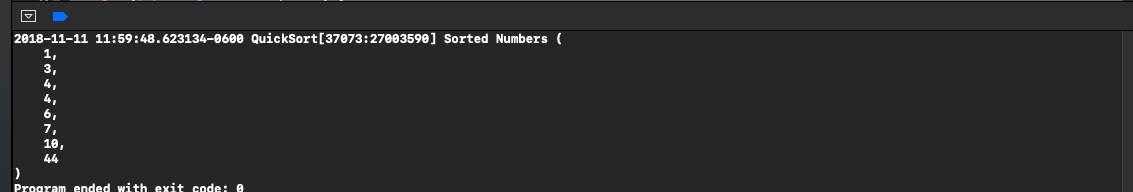# How to Perform a Quicksort in Objective-C

### TagsThe quicksort algorithm is a fundamental sorting algorithm in computer science.  A while back I was looking through my old Objective-C archives and found a quicksort implementation that I had created that never seen the light of day in any project.  To get some use out of this code  and to share this implementation with anyone looking for a quicksort algorithm I thought I would write a brief tutorial on how my implementation of quicksort works in Objective-C.  First, before we get started though there are a few things to keep in mind about this tutorial.  The first is that this tutorial was created as a macOS command line application in Mojave, but any recent version of Xcode or macOS should work with this sample code.  The next is, this tutorial does not require extensive knowledge of Objective-C either, just fundamental Computer Science knowledge.  Let's jump in!

Quicksort in Objective-C 🚀

First, before I dive into the code, let me provide a brief explanation of the quicksort algorithm.  The quicksort algorithm is a sorting algorithm developed by Tony Hoare for dividing data structures up into smaller ones based upon a pivot index.  For example, in the quicksort algorithm, integer arrays are created based upon whether a value in the parent array is greater or less than a chosen pivot index.  Once these arrays are created, they are recursively sorted until only one value remains in each array.  The quicksort algorithm has gained popularity due to it's use in the C standard library and as the Java sorting algorithm behind sort() method.

Below is my recursive implementation of quicksort algorithm in Objective-C.  As mentioned above, the idea is to pick a pivot index.  My implementation uses a pivot index that is based on roughly the middle of the array.  Once that index is obtained, the parent array passed in is used to build three smaller arrays.  One for larger than the pivot, one for smaller than the pivot, and the last is for numbers that equate to the pivot.  This is unique to my implementation, but this was something that I seen to work for duplicate values in the array that equate to the pivot.

The time complexity on this implementation can be as bad as O(n^2), or as good as O(log n).  The space complexity of this implementation is O(log n) based upon how many times the unsortedArray is recursively passed into the method.  ARC should cleanup all of the memory except for what is returned from the quickSortArray: method.

```//
//  main.m
//  QuickSort
//
//  Created by Matt Eaton on 11/11/18.
//

#import <Foundation/Foundation.h>

@interface QuickSort: NSObject

- (NSArray *) quickSortArray:(NSArray *)unsortedArray;
@end

@implementation QuickSort

// ---------- Start of Quick Sort -------------

- (NSArray *) quickSortArray:(NSArray *)unsortedArray {

// Time complexity: O(n^2).
//  * First time arround is to separate the entire array.
//  * Second time around should sort the rest of the array.
// Space complexity: O(log n)  +1 for unsortedArray but the mutable copy never gets added to the autorelease pool.
// All of the arrays that are not attached to the returnArray are released by a compiler pass at the end of the method.

int count = (int)[unsortedArray count];
if (count <= 1) {
return unsortedArray;
}

int pivot = [[unsortedArray objectAtIndex: (count/2)] intValue];
NSMutableArray *smallerThanArray = [NSMutableArray array];
NSMutableArray *largerThanArray = [NSMutableArray array];
NSMutableArray *pivotArray = [NSMutableArray array];

for (int e = 0; e < count; e++) {
int num = [[unsortedArray objectAtIndex:e] intValue];

if (num < pivot) {
} else if (num > pivot) {
// To address the possibly duplicate that is defined in the pivot, in this case 4.
} else if (e != (count/2) && pivot == num) {
}
}

NSMutableArray *returnArray = [NSMutableArray array];

return returnArray;
}

// ---------- End of Quick Sort ---------------

@end

int main(int argc, const char * argv[]) {
@autoreleasepool {

NSArray *quickSortData = @[@4, @3, @10, @44, @6, @4, @1, @7];

QuickSort *qs = [[QuickSort alloc] init];
NSArray *sortedNumbers = [qs quickSortArray:quickSortData];
NSLog(@"Sorted Numbers %@", sortedNumbers);

}
return 0;
}```

The sorted array with the duplicate can be viewed below in the console output.In Summary ⌛️